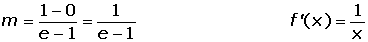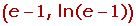## Exercise 1

Calculate the points where the tangent to the curve y = x³ − 3x² − 9x + 5 is parallel to the x-axis.

## Exercise 2

A tangent with a slope of 3 and which passes through the point (0, −2) has been drawn on the curve y= x³. Find the point of contact between the function and the tangent.

## Exercise 3

Find the points on the curve f(x) = x4 + 7x³ + 13x² + x + 1, for which the tangent makes an angle of 45° with the x-axis.

## Exercise 4

Given the function f(x) = tan x, find the angle between the tangent line at the origin of f(x) with the horizontal axis.

## Exercise 5

Calculate the equation of the tangent and normal lines for the curve f(x) = ln tan 2x, at the point where the x-coordinate is: x = π/8.

## Exercise 6

Find the coefficients of the equation y = ax² + bx + c, knowing that its graph passes through (0, 3) and (2, 1), and at the second point, its tangent has a slope of 3.

## Exercise 7

The graph of the function y = ax² + bx + c passes through the points (2, 3) and (3, 13) and the tangent to the curve with an x-coordinate of 1 is parallel to the bisector of the first quadrant. Find the numerical value of a, b and c.

## Exercise 8

Given the function f(x) = ax³ + bx² + cx + d. Determine the value of a, b, c and d knowing that the curve passes through points (−1, 2) (2, 3), and that the tangents at the points on the curve with x-coordinates of 1 and −2 are parallel to the x-axis.

## Exercise 9

At what point of the curve y = ln x, is its tangent parallel to the line that joins the points (1, 0) and (e, 1)?

## Exercise 10

Determine the values of b, for which the tangents to the curve of the function f(x) = b²x³ + bx² + 3x + 9 at x = 1 and x = 2 are parallel.

## Exercise 11

Given the equation 9x²+ y² = 18, find the equation of the tangent line that is parallel to the line of equation 3x − y + 7 = 0.

## Exercise 12

Find the area of the triangle determined by the coordinate axes and the tangent to the curve xy = 1 at x = 1.

## Solution of exercise 1

Calculate the points where the tangent to the curve y = x³ − 3x² − 9x + 5 is parallel to the x-axis.

y' = 3x² − 6x − 9;     x² − 2x − 3 = 0 (simplifying for 3)

x1 = 3 y1 = −22

x2 = −1y2 = 10

A(3, −22) B(−1, 10)

## Solution of exercise 2

A tangent with a slope of 3 and which passes through the point (0, −2) has been drawn on the curve y= x³. Find the point of contact between the function and the tangent.

Point of contact: (a, f(a)).

f'(x)= 3x²f'(a)= 3a²

3a²= 3a = ±1

The equations of the tangent lines are:

a = 1 f(a) = 1

y − 1 = 3(x − 1) y = 3x − 2

a = −1 f(a) = −1

y + 1 = 3(x + 1) y = 3x + 2

The point (0, −2) belongs to the straight line  y = 3x − 2.

So, the point of tangency is (1, 1) .

## Solution of exercise 3

Find the points on the curve f(x) = x4 + 7x³ + 13x² + x + 1, for which the tangent makes an angle of 45° with the x-axis.

m = 1

f'(x) = 4x³ + 21x² + 26x + 1

4x³ + 21x² + 26x + 1 = 1

x = 0 x = −2 x = 13/4

P(0, 4) Q(−2, 4) R(13/4, 1621/256)

## Solution of exercise 4

Given the function f(x) = tan x, find the angle between the tangent line at the origin of f(x) with the horizontal axis.

f′(x) = 1 + tan² x       f′(0) = 1 = m

y = x

α = arc tan 1 = 45º

## Solution of exercise 5

Calculate the equation of the tangent and normal lines for the curve f(x) = ln tan 2x, at the point where the x-coordinate is: x = π/8.Equation of the tangent:Equation of the normal:## Solution of exercise 6

Find the coefficients of the equation y = ax² + bx + c, knowing that its graph passes through (0, 3) and (2, 1), and at the second point, its tangent has a slope of 3.

(0, 3) 3 = c

(2, 1) 1= 4a + 2b + c

y' = 2ax + b 3 = 4a + b

Solving the system:

a = 2 b = −5 c = 3

## Solution of exercise 7

The graph of the function y = ax² + bx + c passes through the points (2, 3) and (3, 13). The tangent to the curve with an x-coordinate of 1 is parallel to the bisector of the first quadrant. Find the numerical value of a, b and c.

(2, 3) 3 = 4a + 2b + c

(3, 13)13 = 9a + 3b +c

y' = 2ax + b 1 = 2a + b

Solve the system:

a = 3 b = −5 c =1

## Solution of exercise 8

Given the function f(x) = ax³ + bx² + cx + d. Determine the value of a, b, c and d knowing that the curve passes through points (−1, 2) (2, 3), and that the tangents at the points on the curve with x-coordinates of 1 and −2 are parallel to the x-axis.

f(−1) = 2 −a + b − c + d = 2

f(2) = 3 8a + 4b + 2c + d = 3

f′(−1) = 0 3a + 2b + c = 0

f′(2) = 0 12a − 4b + c = 0

a = − 2/9 b = − 1/3 c = 4/3 d = 31/9

## Solution of exercise 9

At what point of the curve y = ln x, is its tangent parallel to the line that joins the points (1, 0) and (e, 1)?## Solution of exercise 10

Determine the values of b, for which the tangents to the curve of the function f(x) = b²x³ + bx² + 3x + 9 at x = 1 and x = 2 are parallel.

For both tangent lines to be parallel, the derivatives at x = 1 and x = 2 must be equal.

f'(1) = f'(2)

f'(x) = 3b²x² + 2bx + 3

f'(1) = 3b² + 2b + 3

f'(2) = 12b² + 4b + 3

3b² + 2b + 3 = 12b² + 4b + 3

9b² + 2b = 0

b = 0 b = −2/9

## Solution of exercise 11

Given the equation 9x²+ y²= 18, find the equation of the tangent line that is parallel to the line of equation 3x − y + 7 = 0.

Point of contact: (a, b)

y = 3x + 7             m = 3

After implicitly differentiating there is:## Solution of exercise 12

Find the area of the triangle determined by the coordinate axes and the tangent to the curve xy = 1 at x = 1.Did you like the article?(No Ratings Yet)Loading...

Emma

I am passionate about travelling and currently live and work in Paris. I like to spend my time reading, gardening, running, learning languages and exploring new places.

Did you like
this resource?

Bravo!Download it in pdf format by simply entering your e-mail!

{{ downloadEmailSaved }}

Your email is not valid